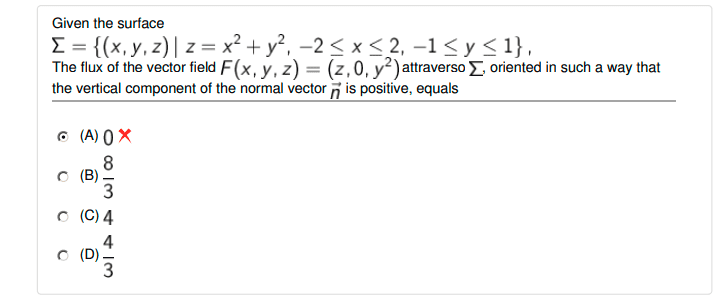# Why can't I use the divergence theorem?

• Amaelle
In summary, the divergence theorem can not be used to solve the problem because the surface is not closed.f

#### Amaelle

Homework Statement
look at the image
Relevant Equations
Divergence theorem
Flux on a surface
Greetings!
here is the following exerciceI understand that when we follow the traditional approach, (prametrization of the surface) we got the answer which is 8/3
But why the divergence theorem can not be used in our case? (I know it's a trap here)
thank you!

•Amaelle
Thanks a million!

•Delta2
You can, however, still use the divergence theorem in solving the problem. If you close the surface by adding a bottom (the rectangle in the xy-plane), the divergence theorem tells you the flux through the top, which is what you're looking for, is equal to the negative of the flux through the bottom, which is easy to calculate.

••Amaelle and FactChecker
thank you but just one point that bothers me, the formula of the Field F (x^2+y^2,0,y^2) doesn't always give 0 gradient?

The field ##\mathbf{F}## has that form only on the surface ##\Sigma##. When you're calculating the general form of the divergence, you need to treat ##x, y,## and ##z## as independent variables.

•Delta2
Yes sorry
I mean F(x,y,z)=(z,0,y^2) and the divergence of F will give 0, this is why for any closed surface this divergence will be 0! this is where I get confused

The divergence theorem tells you that the integral over the closed surface vanishes, so you have
$$\int_{\rm closed~surface} \mathbf{F}\cdot d\vec S = \int_\Sigma \mathbf{F}\cdot d\vec S + \int_{\rm top~surface} \mathbf{F}\cdot d\vec S = 0.$$ Calculate the last integral and note that the first integral has the opposite sign than what the problem asks for.

Last edited:
••Amaelle and Delta2
Amazing! thanks a million!

@vela I think the surface ##\Sigma## is "open" from the above (it doesn't have maximum but minimum at (0,0)), so we actually need a top surface to close it.

Oh, yeah. You’re right. Oops.

•Delta2
I had the wrong picture in my head of the surface ##\Sigma##, so it turns out calculating the total flux through the closed surface has four more pieces corresponding to the sides needed to complete the surface. Because of the symmetry in the problem, those contributions turn out to cancel out, so top+bottom = 0 still holds. But @Amaelle, you should be aware of that since it won't generally be true.

•Amaelle
thank you very much!

@Delta2 , there is a point that confuses me
you said that I couldn't use the Divergence theorem because my surface isn't closed, but isn't the flux over a closed region always =0? thanks!

but isn't the flux over a closed region always =0?
Of course not. It depends on the divergence of the vector field of which we calculate the flux. If the vector field has divergence 0 everywhere then the flux over the closed surface is 0 too, according to divergence theorem. If the divergence is not zero then the flux is equal to the integral of the divergence ##\iiint_V \nabla\cdot \vec{F} dV## where V the volume that is enclosed by the closed surface.

•Amaelle
thanks a million ! you and @vela cleared a huge confusion in my mind!

•vela and Delta2